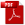Download as PDFAlgebraic relations between certain infinite products
Proceedings of the London Mathematical Society, 2, XVIII, 1920, Records for 13 March 1919

It was proved by Prof. L. J. Rogers1 that \begin{align*} G(x)&= 1+\frac{1}{1-x}+\frac{x^4}{(1-x)(1-x^2)}+ \frac{x^9}{(1-x)(1-x^2)(1-x^3)}+\cdots\\ &= \frac{1}{(1-x)(1-x^6)(1-x^{11})}\cdots \times \frac{1}{(1-x^4)(1-x^9)(1-x^{14})\cdots}, \end{align*} and \begin{align*} H(x)&= 1+\frac{x^2}{1-x}+\frac{x^6}{(1-x)(1-x^2)}+ \frac{x^{12}}{(1-x)(1-x^2)(1-x^3)}+\cdots\\ &= \frac{1}{(1-x^2)(1-x^7)(1-x^{12})}\cdots \times \frac{1}{(1-x^3)(1-x^8)(1-x^{13})\cdots}. \end{align*} Simpler proofs were afterwards found Prof. Rogers and myself.2

I have now found an algebraic relation between $G(x)$ and $H(x)$, viz.: $$H(x)\{G(x)\}^{11}-x^2G(x)\{H(x)\}^{11}=1+11x\{G(x)H(x)\}^6.$$

Another noteworthy formula is $$H(x)G(x^{11})-x^2G(x)H(x^{11})=1.$$ Each of these formul{\ae} is the simplest of a large class.

Endnotes

1Proc. London Math. Soc., Ser. 1, Vol. XXV, 1894, pp. 318 – 343.

2Proc. Camb. Phil. Soc., Vol. XIX, 1919, pp. 211 – 216. A short account of the history of the theorems is given by Mr. Hardy in a note attached to this paper. [For Ramanujan's proofs see No. 26 of this volume.]